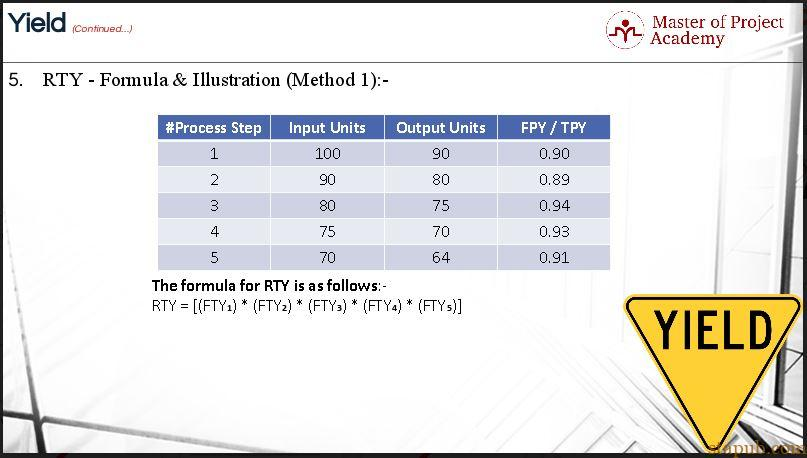A-A+

# FPY与RTY的含义与区别，为什么RTY比FPY更适合

2019年10月09日 阅读 1.3K 人 次

### 为什么RTY比FPY更适合?

• yield的含义及定义
• First-pass yield or Throughput yield (FPY)
• 图解– FPY
• Rolled-throughput Yield (RTY)
• RTY – 公式及图解 (方法 1)
• RTY – 公式及图解(方法 2)

## 两种类型的Yield

### Rolled-throughput Yield (RTY)

RTY小于任何单个过程的最低产量。 每个流程步骤始终具有最小和最大产量。 随着处理步骤数量的增加，RTY指数变小。

## Illustrations of RTY

### First method to calculate RTY

We’ve got 5 process steps in this example. Each step has got input units and output units. Input units are the ones entering each process step, and output units refer to good units produced by each process step. With the help of a formula, we’ve got the first pass yield for each process step. To calculate RTY, multiply the FPY of each process steps to get the answer. In this case, your equation will be the multiplication of five FPY values which will result in 0.6372. In other words, your RTY for all process steps is 63.72%. That was the first throughput yield formula.### Second method to calculate RTY

Let us have a look at the second method of RTY calculation. We’ve again got 5 process steps in this example. To use this method; you need to have two metrics i.e. DPMO and DPU calculated for each process step.

We will now review the calculations to determine the DPU and DPMO.

Calculation of DPU

• Determine the number of units
• Count the number of defects
• Divide the number of defects by the number of units

Calculation of DPMO

• Determine the number of units
• Determine the number of defect opportunities per unit
• Determine the total number of defect opportunities for all the units by multiplying the number of defects per opportunity by the total number of units
• Count the units with defects in the total sample by counting how many opportunities within the sample group contained defects or errors
• Divide the total defects by the total opportunities and multiply by a million

Another simple way to calculate DPU

In this example, we’ve calculated DPU using another quick formula. We’ve divided DPMO figure by 1 million to get DPU for each process step. Why did we do that? DPMO incorporates 1 million defect opportunities, and we need to know defect opportunities for each unit. That is why we divided DPMO by 1 million.

Calculating the RTY from the DPU

In the next column, we have deducted DPU from 1 to get the first-pass yield (FTY) for each process step. The 1 refers to 100%. The logic here is simple. The 100% yield for each process step minus defect opportunities per-unit for each process step is equivalent to good units produced by each process step. In this method, the RTY is calculated by multiplying the FTY for each process as determined in the previous step to get the RTY.

RTY is a realistic view of the yield of any process, looking at all the process steps. The RTY of a process is a good measure of the quality of a process. If the RTY is too low, then a problem-solving team needs to investigate how the process can be improved.